MATLAB Function Referenceconv2

Two-dimensional convolution

Syntax

• ```C = conv2(A,B)
C = conv2(hcol,hrow,A)
C = conv2(...,'shape')
```

Description

```C = conv2(A,B) ``` computes the two-dimensional convolution of matrices `A` and `B`. If one of these matrices describes a two-dimensional finite impulse response (FIR) filter, the other matrix is filtered in two dimensions.

The size of `C` in each dimension is equal to the sum of the corresponding dimensions of the input matrices, minus one. That is, if the size of `A` is `[ma,na]` and the size of `B` is `[mb,nb]`, then the size of `C` is `[ma+mb-1,na+nb-1]`.

```C = conv2(hcol,hrow,A) ``` convolves `A` first with the vector `hcol` along the rows and then with the vector `hrow` along the columns. If `hcol` is a column vector and `hrow` is a row vector, this case is the same as `C = conv2(hcol*hrow,A)`.

```C = conv2(...,'shape') ``` returns a subsection of the two-dimensional convolution, as specified by the `shape` parameter:

 `full` Returns the full two-dimensional convolution (default). `same` Returns the central part of the convolution of the same size as `A`. `valid ` Returns only those parts of the convolution that are computed without the zero-padded edges. Using this option, `C` has size `[ma-mb+1,na-nb+1]` when `all(size(A)` >= `size(B))`. Otherwise `conv2` returns `[]`.

Algorithm

`conv2` uses a straightforward formal implementation of the two-dimensional convolution equation in spatial form. If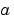and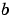are functions of two discrete variables,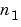and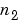, then the formula for the two-dimensional convolution ofandis

•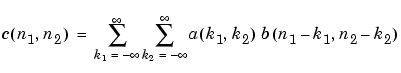In practice however, `conv2` computes the convolution for finite intervals.

Note that matrix indices in MATLAB always start at 1 rather than 0. Therefore, matrix elements `A(1,1)`, `B(1,1)`, and `C(1,1)` correspond to mathematical quantities a(0,0), b(0,0), and c(0,0).

Examples

Example 1. For the `'same'` case, `conv2` returns the central part of the convolution. If there are an odd number of rows or columns, the "center" leaves one more at the beginning than the end.

This example first computes the convolution of A using the default (`'full'`) shape, then computes the convolution using the `'same'` shape. Note that the array returned using `'same'` corresponds to the underlined elements of the array returned using the default shape.

• ```A = rand(3);
B = rand(4);
C = conv2(A,B)           % C is 6-by-6

C =
0.1838  0.2374  0.9727  1.2644  0.7890  0.3750
0.6929  1.2019  1.5499  2.1733  1.3325  0.3096
0.5627  1.5150  2.3576  3.1553  2.5373  1.0602
0.9986  2.3811  3.4302  3.5128  2.4489  0.8462
0.3089  1.1419  1.8229  2.1561  1.6364  0.6841
0.3287  0.9347  1.6464  1.7928  1.2422  0.5423

Cs = conv2(A,B,'same')   % Cs is the same size as A: 3-by-3
Cs =
2.3576  3.1553  2.5373
3.4302  3.5128  2.4489
1.8229  2.1561  1.6364
```

Example 2. In image processing, the Sobel edge finding operation is a two-dimensional convolution of an input array with the special matrix

• ```s = [1 2 1; 0 0 0; -1 -2 -1];
```

These commands extract the horizontal edges from a raised pedestal.

• ```A = zeros(10);
A(3:7,3:7) = ones(5);
H = conv2(A,s);
mesh(H)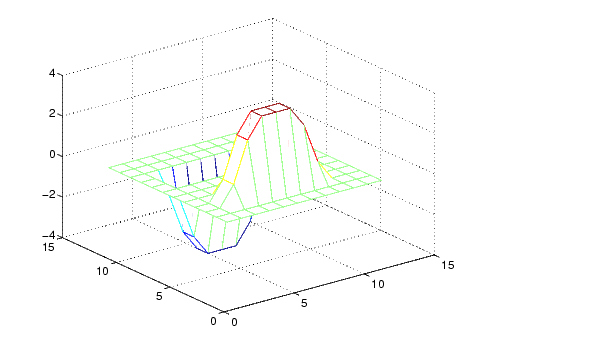```

Transposing the filter `s` extracts the vertical edges of `A`.

• ```V = conv2(A,s');
figure, mesh(V)```

This figure combines both horizontal and vertical edges.

• ```figure
mesh(sqrt(H.^2 + V.^2))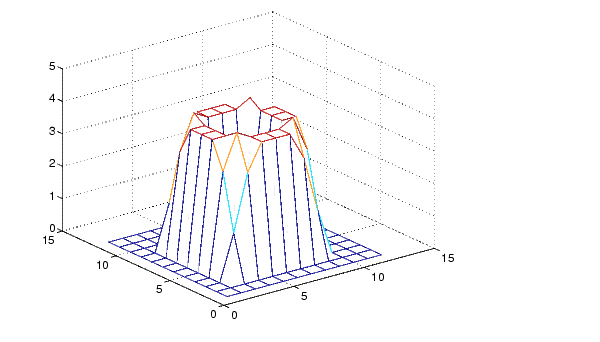```

See Also

`conv`, `convn`, `filter2`

`xcorr2` in the Signal Processing Toolbox

© 1994-2005 The MathWorks, Inc.